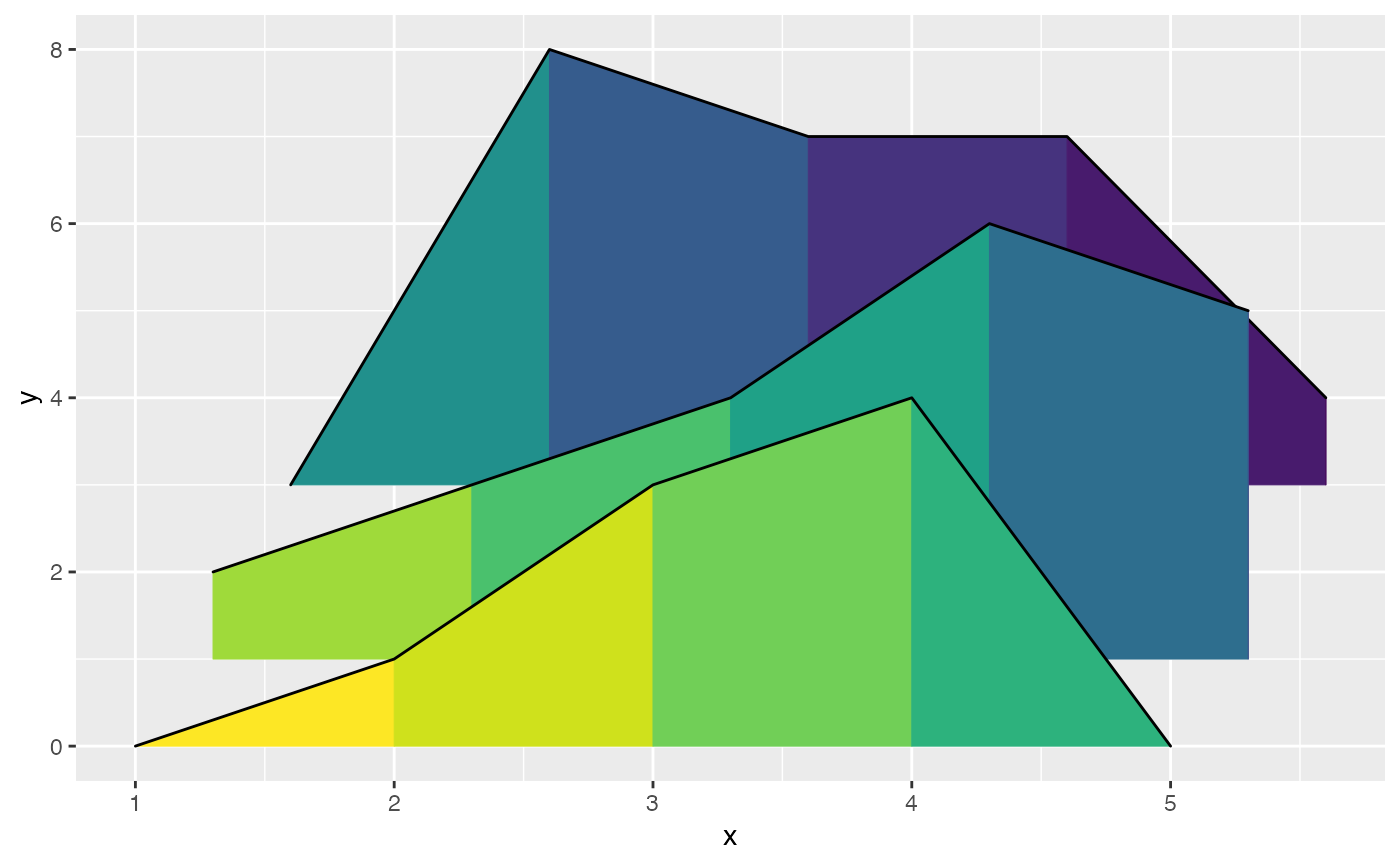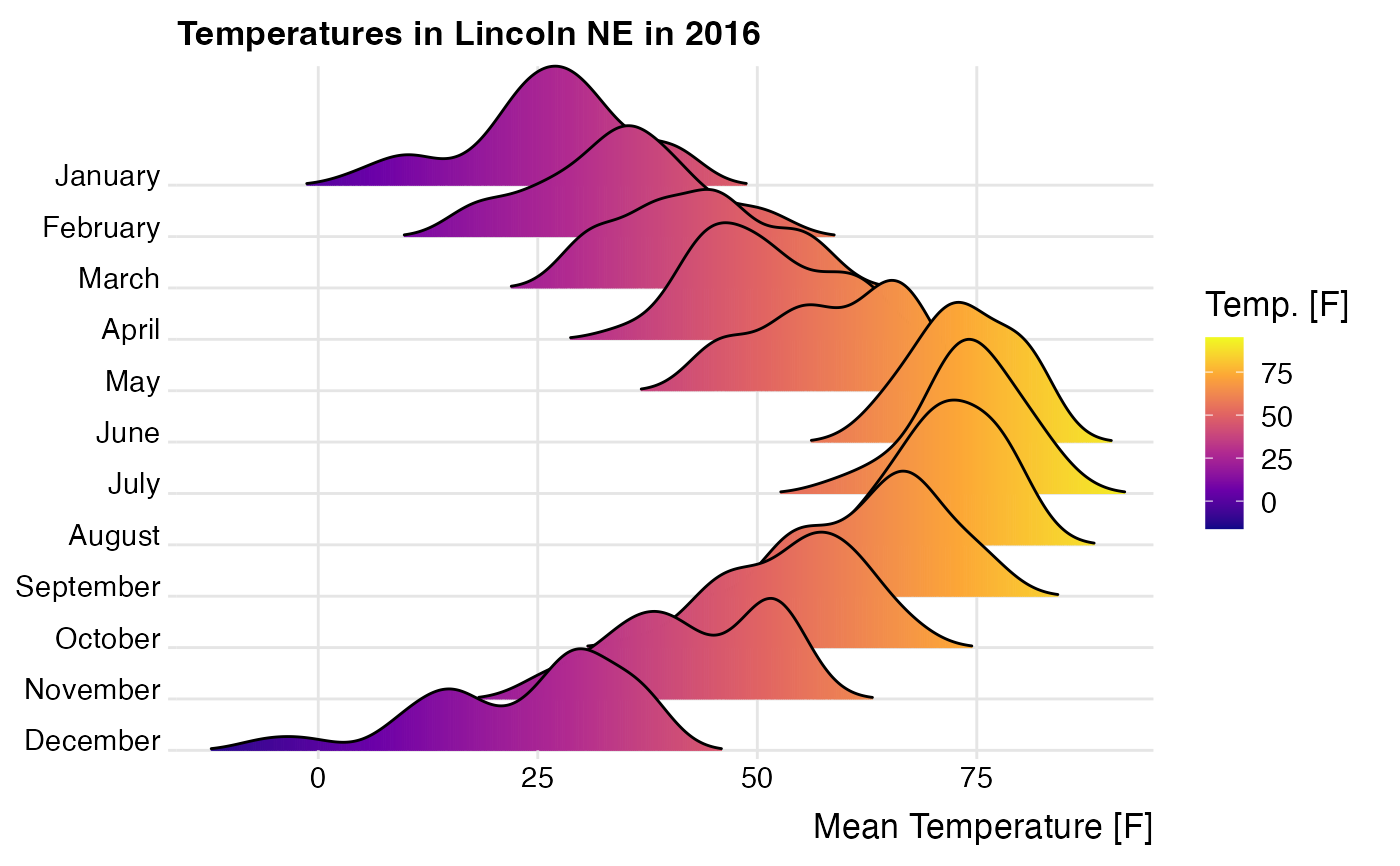The geoms geom_ridgeline_gradient and geom_density_ridges_gradient work just like geom_ridgeline and geom_density_ridges except that the fill aesthetic can vary along the x axis. Because filling with color gradients is fraught with issues, these geoms should be considered experimental. Don't use them unless you really need to. Note that due to limitations in R's graphics system, transparency (alpha) has to be disabled for gradient fills.

geom_ridgeline_gradient(
mapping = NULL,
data = NULL,
stat = "identity",
position = "identity",
na.rm = FALSE,
show.legend = NA,
inherit.aes = TRUE,
...
)

mapping = NULL,
data = NULL,
stat = "density_ridges",
position = "points_sina",
panel_scaling = TRUE,
na.rm = TRUE,
show.legend = NA,
inherit.aes = TRUE,
...
)

## Arguments

mapping Set of aesthetic mappings created by aes() or aes_(). If specified and inherit.aes = TRUE (the default), it is combined with the default mapping at the top level of the plot. You must supply mapping if there is no plot mapping. The data to be displayed in this layer. There are three options: If NULL, the default, the data is inherited from the plot data as specified in the call to ggplot(). A data.frame, or other object, will override the plot data. A function will be called with a single argument, the plot data. The return value must be a data.frame., and will be used as the layer data. The statistical transformation to use on the data for this layer, as a string. Position adjustment, either as a string, or the result of a call to a position adjustment function. If FALSE, the default, missing values are removed with a warning. If TRUE, missing values are silently removed. A parameter to needed to remove rendering artifacts inside the rendered gradients. Should ideally be 0, but often needs to be around 0.5 or higher. logical. Should this layer be included in the legends? NA, the default, includes if any aesthetics are mapped. FALSE never includes, and TRUE always includes. If FALSE, overrides the default aesthetics, rather than combining with them. other arguments passed on to layer(). These are often aesthetics, used to set an aesthetic to a fixed value, like color = "red" or size = 3. They may also be parameters to the paired geom/stat. Argument only to geom_density_ridges_gradient. If TRUE, the default, relative scaling is calculated separately for each panel. If FALSE, relative scaling is calculated globally.

## Examples

library(ggplot2)

# Example for geom_ridgeline_gradient()
d <- data.frame(
x = rep(1:5, 3) + c(rep(0, 5), rep(0.3, 5), rep(0.6, 5)),
y = c(rep(0, 5), rep(1, 5), rep(3, 5)),
height = c(0, 1, 3, 4, 0, 1, 2, 3, 5, 4, 0, 5, 4, 4, 1)
)
ggplot(d, aes(x, y, height = height, group = y, fill = factor(x+y))) +
scale_fill_viridis_d(direction = -1) +
theme(legend.position = 'none')# Example for geom_density_ridges_gradient()
ggplot(lincoln_weather, aes(x = Mean Temperature [F], y = Month, fill = stat(x))) +
geom_density_ridges_gradient(scale = 3, rel_min_height = 0.01) +
scale_x_continuous(expand = c(0, 0)) +
scale_y_discrete(expand = c(0, 0)) +
scale_fill_viridis_c(name = "Temp. [F]", option = "C") +
coord_cartesian(clip = "off") +
labs(title = 'Temperatures in Lincoln NE in 2016') +
theme_ridges(font_size = 13, grid = TRUE) +
theme(axis.title.y = element_blank())
#> Picking joint bandwidth of 3.37